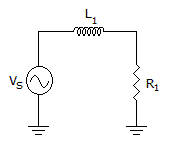# Electronics - RL Circuits - Discussion

### Discussion :: RL Circuits - Filling the Blanks (Q.No.1)

1.If the resistance is larger than the inductive reactance in the given circuit, the phase angle is _______.

 [A]. less than 0° [B]. between 0° and 45° [C]. between 45° and 0° [D]. greater than 90°

Explanation:

No answer description available for this question.

 Deepak said: (Jan 27, 2012) angle is tan^-1=xL/r, r<1forangle0-45

 Chandan said: (Aug 20, 2012) Let us assume resistance is infinity than tan-(xl/infinity) that is tan-(0) so it is zero. and in second case assume r=xl than tan-(1) that is 45 degree. So answer is 0degree and 45degree

 Asma Asghar Janjua said: (Jun 18, 2016) z^2 = r^2 + xl^2. According to Pythagoras theorem, h^2 = b^2 + p^2. angle = tan^-1(xl/r). Then, we get the answer.